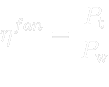## Fan Efficiency• Pt    aerodynamic power,[W]
• Pw    torque power,[W]
• f     compress factor,[-]
• Yst     static work,[m/s]
• Yd    dynamic work,[m/s]
• Yt    total work,[m/s]
• psi    pressure number,[-]
• phi    flow number,[-]
• axialForce    axial force on rotor,[N]
• pTotInlet     total pressure at the inlet,[Pa]
• pTotVolute     total pressure at the outlet,[Pa]
• pTotOutlet     total pressure at the wheel outlet,[Pa]
• pInlet     static pressure at the inlet,[Pa]
• pVolute     static pressure at the outlet,[Pa]
• pOutlet     static pressure at the wheel outlet,[Pa]
• magUInlet     velocity at the inlet,[m/s]
• magUVolute     velocity at the outlet,[m/s]
• magUOutlet     velocity at the wheel outlet,[m/s]
• massFlowInlet     mass flow at the inlet,[kg/s]
• massFlowVolute     mass flow at the outlet,[kg/s]
• massFlowOutlet     mass flow at the wheel outlet,[kg/s]
• rhoInlet     density at the inlet,[kg/m]
• rhoVolute         density at the outlet,[kg/m]
• rhoOutlet     density at the wheel outlet,[kg/m]
• volumeFlowRateInlet     volumetric flow rate at the inlet,[m/s]
• volumeFlowRateVolute     volumetric flow rate at the outlet,[m/s]
• volumeFlowRateOutlet     volumetric flow rate at the wheel outlet,[m/s]
• moment     torque at wheel,[Nm]
• totalPressureDifference     difference in total pressure inlet-outlet,[Pa]
• staticPressureDifference     difference in static pressure inlet-outlet,[Pa]